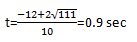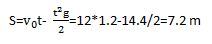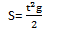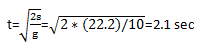76 248
Assignments Done
98,5%
Successfully Done
In May 2019

# Answer to Question #5127 in Mechanics | Relativity for Kristen

Question #5127
Two rocks are thrown off the edge of a cliff that is 15.0 m above the ground. The first rock is thrown upward, at a velocity of +12.0 m/s. The second is thrown downward, at a velocity of −12.0 m/s. Ignore air resistance. Determine (a) how long it takes the first rock to hit the ground and (b) at what velocity it hits. Determine (c) how long it takes the second rock to hit the ground and (d) at what velocity it hits.
Expert's answer
a) S=[img width=14,height=20]file:///C:/Users/Sekventa/AppData/Local/Temp/OICE_B5A94EB8-5E00-4A5C-AF4E-5C45A3C9F209.0/msohtmlclip1/01/clip_image002.gif[/img]V0t+gt^2/2
15=12t+5t^2
5t^2+12t-15=0b)
V=V0+gt=12+0.9*10=21m/s
c) V0=gt
t=V0/g=1.2 s it flies upwardsand againall time is equal to sum 2.1 sec+ 1.2 sec =3.3sec
d)V=V0+gt here V0=0
V=gt=3.3*10=33m/s

Need a fast expert's response?

Submit order

and get a quick answer at the best price

for any assignment or question with DETAILED EXPLANATIONS!

#### Comments

No comments. Be first!

Submit